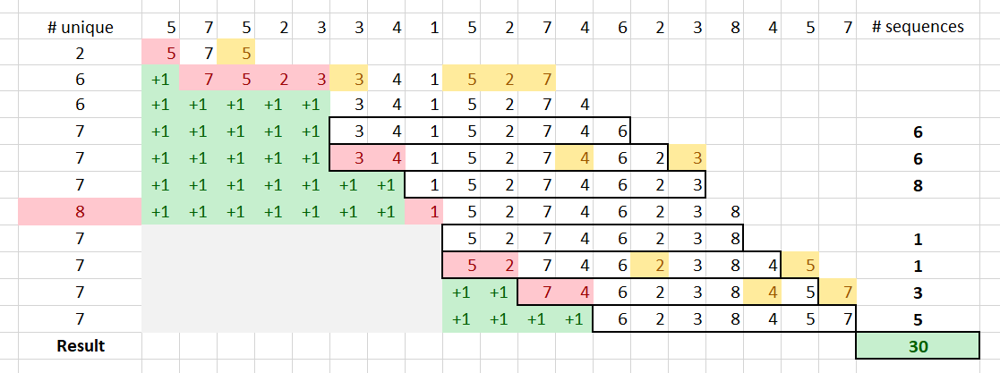## Sunday, February 10, 2019

### Interview Pearls: Sliding Window

If the problem talks about continuous subarrays or substrings, the sliding window technique may help solve it in a linear time. Such problems are tricky, though the solution is simple once you get it. No wonder I am seeing such problems in almost every interview!
Here, we will take a look at Subarrays with K Different Integers (LeetCode Hard), which appeared on LeetCode weekly contest #123. You can also master the sliding windows technique with these additional problems:

# Problem Description

Given an array `A` of positive integers, call a (contiguous, not necessarily distinct) subarray of `A` good if the number of different integers in that subarray is exactly `K`. For example, `[1,2,3,1,2]` has 3 different integers: 1, 2, and 3.
Return the number of good subarrays of `A`.

### Example

Input: A = [1,2,1,2,3], K = 2
Output: 7
Explanation: Subarrays formed with exactly 2 different integers: [1,2], [2,1], [1,2], [2,3], [1,2,1], [2,1,2], [1,2,1,2].

# Coding Practice

Try solving this problem before moving on to the solutions. It is available on LeetCode Online Judge (Subarrays with K Different Integers). Also, as you read through a solution, try implementing it yourself.
LeetCode is my favorite destinations for algorithmic problems. It has 987 problems and counting, with test cases to validate the correctness, as well as computational and memory complexity. There is also a large community discussing different approaches, tips and tricks.

# Brute-Force Solution

We can just iterate through each sub-array using two loops and count the good ones. For the inner loop, we use hash set to count unique numbers; if we the set size becomes larger than `K`, we break from the inner loop.
``````int subarraysWithKDistinct(vector<int>& A, int K, int res = 0) {
for (auto i = 0; i < A.size(); ++i) {
unordered_set<int> s;
for (auto j = i; j < A.size() && s.size() <= K; ++j) {
s.insert(A[j]);
if (s.size() == K) ++res;
}
}
return res;
}
``````

### Complexity Analysis

The time complexity of this solution is O(n * n), where n is the length of `A`. This solution is not accepted by the online judge.

# Intuition

If the subarray `[j, i]` contains `K` unique numbers, and first `prefix` numbers also appear in `[j + prefix, i]` subarray, we have total `1 + prefix` good subarrays. For example, there are 3 unique numers in `[1, 2, 1, 2, 3]`. First two numbers also appear in the remaining subarray `[1, 2, 3]`, so we have 1 + 2 good subarrays: `[1, 2, 1, 2, 3]``[2, 1, 2, 3]` and `[1, 2, 3]`.

# Linear Solution

We can iterate through the array and use two pointers for our sliding window (`[j, i]`). The back of the window is always the current position in the array (`i`). The front of the window (`j`) is moved so that A[j] appear only once in the sliding window. In other words, we are trying to shrink our sliding window while maintaining the same number of unique elements.
To do that, we keep tabs on how many times each number appears in our window (`m`). After we add next number to the back of our window, we try to remove as many as possible numbers from the front, until the number in the front appears only once. While removing numbers, we are increasing `prefix`.
If we collected `K` unique numbers, then we found 1 + `prefix` sequences, as each removed number would also form a sequence.
If our window reached `K + 1` unique numbers, we remove one number from the head (again, that number appears only in the front), and reset `prefix` as now we are starting a new sequence. This process is demonstrated step-by-step for the test case below; `prefix` are shown as `+1` in the green background.
``````[5,7,5,2,3,3,4,1,5,2,7,4,6,2,3,8,4,5,7]
7
``````In the code below, we use `cnt` to track unique numbers. Since `1 <= A[i] <= A.size()`, we can use an array instead of hash map to improve the performance.
``````int subarraysWithKDistinct(vector<int>& A, int K, int res = 0) {
vector<int> m(A.size() + 1);
for(auto i = 0, j = 0, prefix = 0, cnt = 0; i < A.size(); ++i) {
if (m[A[i]]++ == 0) ++cnt;
if (cnt > K) --m[A[j++]], --cnt, prefix = 0;
while (m[A[j]] > 1) ++prefix, --m[A[j++]];
if (cnt == K) res += prefix + 1;
}
return res;
}
``````
Java version:
``````public int subarraysWithKDistinct(int[] A, int K) {
int res = 0, prefix = 0;
int[] m = new int[A.length + 1];
for (int i = 0, j = 0, cnt = 0; i < A.length; ++i) {
if (m[A[i]]++ == 0) ++cnt;
if (cnt > K) {
--m[A[j++]]; --cnt; prefix = 0;
}
while (m[A[j]] > 1) {
++prefix; --m[A[j++]];
}
if (cnt == K) res += prefix + 1;
}
return res;
}
``````

### Complexity Analysis

• Time Complexity: O(n), where n is the length of `A`.
• Space Complexity: O(n).

1.Very nice and intuitive explanation. I never thought that I can include the logic of prefix into a sliding window problem. Could you let me know what led you to this thought process?

2.It can be easily solved using the fact you can calculate number of subarrays with <K and with <=K distinct elements. Which is very close to what you have described.

3.can u explain by taking days,week,month data using sliding window concept sir....
Eg: w1=(d1=40,d2=30,d3=42,d4=30,d5=60,d6=80,d7=120)..........

4.You are doing a great job by writing such informative article. Interesting at the same time. Also check this out Cheap glass fencing gold coast. Thank you.

5.I never realized there were so many different types of wall planter available! Your post opened my eyes to a whole new world of possibilities.